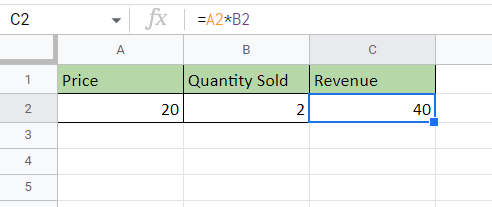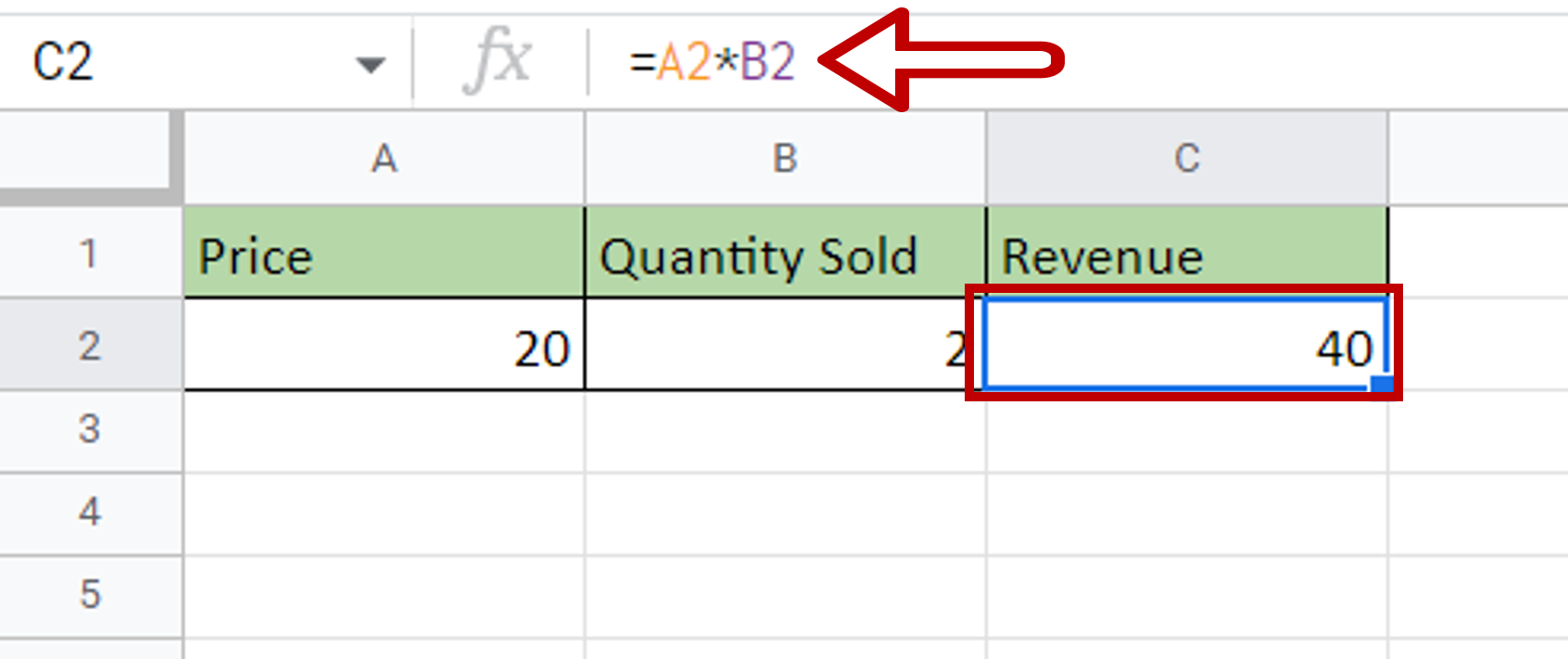# How to multiply two cells in Google Sheets

You can watch a video tutorial here.Google Sheets is widely used for calculations due to the several arithmetic operators and functions that it has. You may need to multiply two cells, for example, if you have the Price and Quantity sold and you need to compute the Revenue.

### Step 1 – Select the destination– Select the cell in which the result is to be displayed

### Step 2 – Multiply the cells– Type the formula using cell references:
=Price* Quantity Sold
– Press Enter

### Step 3 – Check the result– The cells are multiplied and the result is displayed in the destination cell